Submitted Successfully!
Thank you for your contribution! You can also upload a video entry or images related to this topic.
Ver. Summary Created by Modification Content Size Created at Operation
1 handwiki -- 2535 2022-10-08 05:41:59

Do you have a full video?

# Confirm

Are you sure to Delete?
Cite
Liu, H. Nontransitive Dice. Encyclopedia. Available online: https://encyclopedia.pub/entry/36846 (accessed on 09 December 2023).
Liu H. Nontransitive Dice. Encyclopedia. Available at: https://encyclopedia.pub/entry/36846. Accessed December 09, 2023.
Liu, Handwiki. "Nontransitive Dice" Encyclopedia, https://encyclopedia.pub/entry/36846 (accessed December 09, 2023).
Liu, H.(2022, November 28). Nontransitive Dice. In Encyclopedia. https://encyclopedia.pub/entry/36846
Liu, Handwiki. "Nontransitive Dice." Encyclopedia. Web. 28 November, 2022.
Nontransitive Dice

A set of dice is nontransitive if it contains three dice, A, B, and C, with the property that A rolls higher than B more than half the time, and B rolls higher than C more than half the time, but it is not true that A rolls higher than C more than half the time. In other words, a set of dice is nontransitive if the binary relation – X rolls a higher number than Y more than half the time – on its elements is not transitive. It is possible to find sets of dice with the even stronger property that, for each die in the set, there is another die that rolls a higher number than it more than half the time. Using such a set of dice, one can invent games which are biased in ways that people unused to nontransitive dice might not expect (see Example).

binary relation dice nontransitive

## 1. Example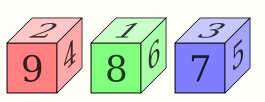An example of nontransitive dice (opposite sides have the same value as those shown). https://handwiki.org/wiki/index.php?curid=1997326

Consider the following set of dice.

• Die A has sides 2, 2, 4, 4, 9, 9.
• Die B has sides 1, 1, 6, 6, 8, 8.
• Die C has sides 3, 3, 5, 5, 7, 7.

The probability that A rolls a higher number than B, the probability that B rolls higher than C, and the probability that C rolls higher than A are all 5/9, so this set of dice is nontransitive. In fact, it has the even stronger property that, for each die in the set, there is another die that rolls a higher number than it more than half the time.

Now, consider the following game, which is played with a set of dice.

1. The first player chooses a die from the set.
2. The second player chooses one die from the remaining dice.
3. Both players roll their die; the player who rolls the higher number wins.

If this game is played with a transitive set of dice, it is either fair or biased in favor of the first player, because the first player can always find a die that will not be beaten by any other dice more than half the time. If it is played with the set of dice described above, however, the game is biased in favor of the second player, because the second player can always find a die that will beat the first player's die with probability 5/9. The following tables show all possible outcomes for all 3 pairs of dice.

 2 4 9 1 6 8 3 A B C C A B Player 1 chooses die APlayer 2 chooses die C Player 1 chooses die BPlayer 2 chooses die A Player 1 chooses die CPlayer 2 chooses die B C A A A B B C C C C C A A B B B B C C C A A A A B B B

## 2. Comment Regarding the Equivalency of Nontransitive Dice

Though the three nontransitive dice A, B, C (first set of dice)

• A: 2, 2, 6, 6, 7, 7
• B: 1, 1, 5, 5, 9, 9
• C: 3, 3, 4, 4, 8, 8

P(A > B) = P(B > C) = P(C > A) = 5/9

and the three nontransitive dice A′, B′, C′ (second set of dice)

• A′: 2, 2, 4, 4, 9, 9
• B′: 1, 1, 6, 6, 8, 8
• C′: 3, 3, 5, 5, 7, 7

P(A′ > B′) = P(B′ > C′) = P(C′ > A′) = 5/9

win against each other with equal probability they are not equivalent. While the first set of dice (A, B, C) has a 'highest' die, the second set of dice has a 'lowest' die. Rolling the three dice of a set and using always the highest score for evaluation will show a different winning pattern for the two sets of dice. With the first set of dice, die B will win with the highest probability (88/216) and dice A and C will each win with a probability of 64/216. With the second set of dice, die C′ will win with the lowest probability (56/216) and dice A′ and B′ will each win with a probability of 80/216.

## 3. Variations

### 3.1. Efron's Dice

Efron's dice are a set of four nontransitive dice invented by Bradley Efron.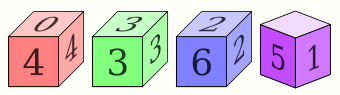Representation of Efron's dice. https://handwiki.org/wiki/index.php?curid=2021619

The four dice A, B, C, D have the following numbers on their six faces:

• A: 4, 4, 4, 4, 0, 0
• B: 3, 3, 3, 3, 3, 3
• C: 6, 6, 2, 2, 2, 2
• D: 5, 5, 5, 1, 1, 1

#### Probabilities

Each die is beaten by the previous die in the list, with a probability of 2/3:

$\displaystyle{ P(A\gt B) = P(B\gt C) = P(C\gt D) = P(D\gt A) = {2 \over 3} }$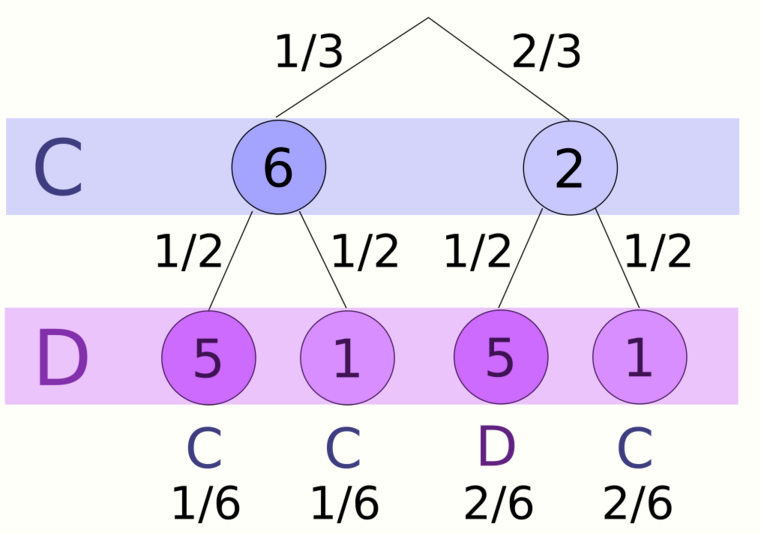A conditional probability tree can be used to discern the probability with which C rolls higher than D. https://handwiki.org/wiki/index.php?curid=2021652

B's value is constant; A beats it on 2/3 rolls because four of its six faces are higher.

Similarly, B beats C with a 2/3 probability because only two of C's faces are higher.

P(C>D) can be calculated by summing conditional probabilities for two events:

• C rolls 6 (probability 1/3); wins regardless of D (probability 1)
• C rolls 2 (probability 2/3); wins only if D rolls 1 (probability 1/2)

The total probability of win for C is therefore

$\displaystyle{ \left( {1 \over 3}\times1 \right) + \left( {2 \over 3}\times{1 \over 2} \right) = {2 \over 3} }$

With a similar calculation, the probability of D winning over A is

$\displaystyle{ \left( {1 \over 2}\times1 \right) + \left( {1 \over 2}\times{1 \over 3} \right) = {2 \over 3} }$

#### Best overall die

The four dice have unequal probabilities of beating a die chosen at random from the remaining three:

As proven above, die A beats B two-thirds of the time but beats D only one-third of the time. The probability of die A beating C is 4/9 (A must roll 4 and C must roll 2). So the likelihood of A beating any other randomly selected die is:

$\displaystyle{ {1 \over 3}\times \left( {2 \over 3} + {1 \over 3} + {4 \over 9} \right) = {13 \over 27} }$

Similarly, die B beats C two-thirds of the time but beats A only one-third of the time. The probability of die B beating D is 1/2 (only when D rolls 1). So the likelihood of B beating any other randomly selected die is:

$\displaystyle{ {1 \over 3}\times \left( {2 \over 3} + {1 \over 3} + {1 \over 2} \right) = {1 \over 2} }$

Die C beats D two-thirds of the time but beats B only one-third of the time. The probability of die C beating A is 5/9. So the likelihood of C beating any other randomly selected die is:

$\displaystyle{ {1 \over 3}\times \left( {2 \over 3} + {1 \over 3} + {5 \over 9} \right) = {14 \over 27} }$

Finally, die D beats A two-thirds of the time but beats C only one-third of the time. The probability of die D beating B is 1/2 (only when D rolls 5). So the likelihood of D beating any other randomly selected die is:

$\displaystyle{ {1 \over 3}\times \left( {2 \over 3} + {1 \over 3} + {1 \over 2} \right) = {1 \over 2} }$

Therefore, the best overall die is C with a probability of winning of 0.5185. C also rolls the highest average number in absolute terms, 3+1/3. (A's average is 2+2/3, while B's and D's are both 3.)

#### Variants with equal averages

Note that Efron's dice have different average rolls: the average roll of A is 8/3, while B and D each average 9/3, and C averages 10/3. The nontransitive property depends on which faces are larger or smaller, but does not depend on the absolute magnitude of the faces. Hence one can find variants of Efron's dice where the odds of winning are unchanged, but all the dice have the same average roll. For example,

• A: 7, 7, 7, 7, 1, 1
• B: 5, 5, 5, 5, 5, 5
• C: 9, 9, 3, 3, 3, 3
• D: 8, 8, 8, 2, 2, 2

These variant dice are useful, e.g., to introduce students to different ways of comparing random variables (and how only comparing averages may overlook essential details).

### Numbered 1 through 24 dice

A set of four dice using all of the numbers 1 through 24 can be made to be nontransitive. With adjacent pairs, one die's probability of winning is 2/3.

For rolling high number, B beats A, C beats B, D beats C, A beats D.

• A: 01, 02, 16, 17, 18, 19
• B: 03, 04, 05, 20, 21, 22
• C: 06, 07, 08, 09, 23, 24
• D: 10, 11, 12, 13, 14, 15

#### Relation to Efron's dice

These dice are basically the same as Efron's dice, as each number of a series of successive numbers on a single die can all be replaced by the lowest number of the series and afterwards renumbering them.

• A: 01, 02, 16, 17, 18, 1901, 01, 16, 16, 16, 160, 0, 4, 4, 4, 4
• B: 03, 04, 05, 20, 21, 2203, 03, 03, 20, 20, 201, 1, 1, 5, 5, 5
• C: 06, 07, 08, 09, 23, 2406, 06, 06, 06, 23, 232, 2, 2, 2, 6, 6
• D: 10, 11, 12, 13, 14, 1510, 10, 10, 10, 10, 103, 3, 3, 3, 3, 3

### Miwin's dice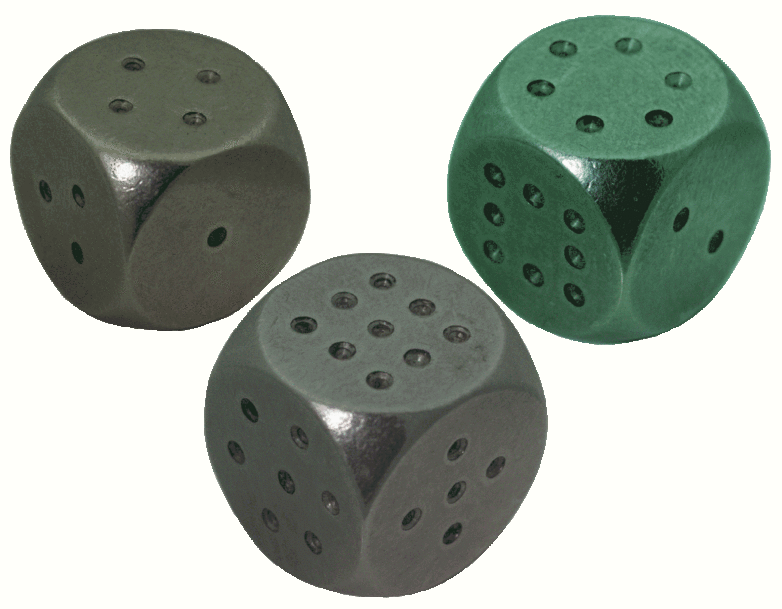Miwin's dice. https://handwiki.org/wiki/index.php?curid=1579160

Miwin's Dice were invented in 1975 by the physicist Michael Winkelmann.

Consider a set of three dice, III, IV and V such that

• die III has sides 1, 2, 5, 6, 7, 9
• die IV has sides 1, 3, 4, 5, 8, 9
• die V has sides 2, 3, 4, 6, 7, 8

Then:

• the probability that III rolls a higher number than IV is 17/36
• the probability that IV rolls a higher number than V is 17/36
• the probability that V rolls a higher number than III is 17/36

### Three-dice set with minimal alterations to standard dice

The following nontransitive dice have only a few differences compared to 1 through 6 standard dice:

• as with standard dice, the total number of pips is always 21
• as with standard dice, the sides only carry pip numbers between 1 and 6
• faces with the same number of pips occur a maximum of twice per dice
• only two sides on each die have numbers different from standard dice:
• A: 1, 1, 3, 5, 5, 6
• B: 2, 3, 3, 4, 4, 5
• C: 1, 2, 2, 4, 6, 6

Like Miwin’s set, the probability of A winning versus B (or B vs. C, C vs. A) is 17/36. The probability of a draw, however, is 4/36, so that only 15 out of 36 rolls lose. So the overall winning expectation is higher.

## 4. Warren Buffett

Warren Buffett is known to be a fan of nontransitive dice. In the book Fortune's Formula: The Untold Story of the Scientific Betting System that Beat the Casinos and Wall Street, a discussion between him and Edward Thorp is described. Buffett and Thorp discussed their shared interest in nontransitive dice. "These are a mathematical curiosity, a type of 'trick' dice that confound most people's ideas about probability."

Buffett once attempted to win a game of dice with Bill Gates using nontransitive dice. "Buffett suggested that each of them choose one of the dice, then discard the other two. They would bet on who would roll the highest number most often. Buffett offered to let Gates pick his die first. This suggestion instantly aroused Gates's curiosity. He asked to examine the dice, after which he demanded that Buffett choose first."

In 2010, Wall Street Journal magazine quoted Sharon Osberg, Buffett's bridge partner, saying that when she first visited his office 20 years earlier, he tricked her into playing a game with nontransitive dice that could not be won and "thought it was hilarious".

## 5. Nontransitive Dice Set for More Than Two Players

A number of people have introduced variations of nontransitive dice where one can compete against more than one opponent.

### Three players

#### Oskar dice

Oskar van Deventer introduced a set of seven dice (all faces with probability 1/6) as follows:

• A: 2, 02, 14, 14, 17, 17
• B: 7, 07, 10, 10, 16, 16
• C: 5, 05, 13, 13, 15, 15
• D: 3, 03, 09, 09, 21, 21
• E: 1, 01, 12, 12, 20, 20
• F: 6, 06, 08, 08, 19, 19
• G: 4, 04, 11, 11, 18, 18

One can verify that A beats {B,C,E}; B beats {C,D,F}; C beats {D,E,G}; D beats {A,E,F}; E beats {B,F,G}; F beats {A,C,G}; G beats {A,B,D}. Consequently, for arbitrarily chosen two dice there is a third one that beats both of them. Namely,

• G beats {A,B}; F beats {A,C}; G beats {A,D}; D beats {A,E}; D beats {A,F}; F beats {A,G};
• A beats {B,C}; G beats {B,D}; A beats {B,E}; E beats {B,F}; E beats {B,G};
• B beats {C,D}; A beats {C,E}; B beats {C,F}; F beats {C,G};
• C beats {D,E}; B beats {D,F}; C beats {D,G};
• D beats {E,F}; C beats {E,G};
• E beats {F,G}.

Whatever the two opponents choose, the third player will find one of the remaining dice that beats both opponents' dice.

#### Grime dice

Dr. James Grime discovered a set of five dice as follows:

• A: 2, 2, 2, 7, 7, 7
• B: 1, 1, 6, 6, 6, 6
• C: 0, 5, 5, 5, 5, 5
• D: 4, 4, 4, 4, 4, 9
• E: 3, 3, 3, 3, 8, 8

One can verify that, when the game is played with one set of Grime dice:

• A beats B beats C beats D beats E beats A (first chain);
• A beats C beats E beats B beats D beats A (second chain).

However, when the game is played with two such sets, then the first chain remains the same (with one exception discussed later) but the second chain is reversed (i.e. A beats D beats B beats E beats C beats A). Consequently, whatever dice the two opponents choose, the third player can always find one of the remaining dice that beats them both (as long as the player is then allowed to choose between the one-die option and the two-die option):

Sets chosen
by opponents
Winning set of dice
Type Number
A B E 1
A C E 2
A D C 2
A E D 1
B C A 1
B D A 2
B E D 2
C D B 1
C E B 2
D E C 1

There are two major issues with this set, however. The first one is that in the two-die option of the game, the first chain should stay exactly the same in order to make the game nontransitive. In practice, though, D actually beats C. The second problem is that the third player would have to be allowed to choose between the one-die option and the two-die option – which may be seen as unfair to other players.

##### Corrected Grime dice

The above issue of D defeating C arises because the dice have 6 faces rather than 5. By replacing the lowest (or highest) face of each die with "reroll" (R), all five dice will function exactly as Dr. James Grime intended:

• A: R, 2, 2, 7, 7, 7
• B: R, 1, 6, 6, 6, 6
• C: R, 5, 5, 5, 5, 5
• D: R, 4, 4, 4, 4, 9
• E: R, 3, 3, 3, 8, 8

Alternatively, these faces could be mapped to a set of pentagonal-trapezohedral (10-sided) dice, with each number appearing exactly twice, or to a set of icosahedral (20-sided) dice, with each number appearing four times. This eliminates the need for a "reroll" face.

This solution was discovered by Jon Chambers, an Australian Pre-Service Mathematics Teacher.

### Four players

A four-player set has not yet been discovered, but it was proved that such a set would require at least 19 dice.

## 6. Nontransitive 4-sided Dice

Tetrahedra can be used as dice with four possible results.

Set 1
• A: 1, 4, 7, 7
• B: 2, 6, 6, 6
• C: 3, 5, 5 ,8

P(A > B) = P(B > C) = P(C > A) = 9/16

The following tables show all possible outcomes:

B
A
2 6 6 6
1 B B B B
4 A B B B
7 A A A A
7 A A A A

In "A versus B", A wins in 9 out of 16 cases.

C
B
3 5 5 8
2 C C C C
6 B B B C
6 B B B C
6 B B B C

In "B versus C", B wins in 9 out of 16 cases.

A
C
1 4 7 7
3 C A A A
5 C C A A
5 C C A A
8 C C C C

In "C versus A", C wins in 9 out of 16 cases.

Set 2
• A: 3, 3, 3, 6
• B: 2, 2, 5, 5
• C: 1, 4, 4, 4

P(A > B) = P(B > C) = 10/16, P(C > A) = 9/16

## 7. Nontransitive 12-sided Dice

In analogy to the nontransitive six-sided dice, there are also dodecahedra which serve as nontransitive twelve-sided dice. The points on each of the dice result in the sum of 114. There are no repetitive numbers on each of the dodecahedra.

Miwin’s dodecahedra (set 1) win cyclically against each other in a ratio of 35:34.

The miwin’s dodecahedra (set 2) win cyclically against each other in a ratio of 71:67.

Set 1: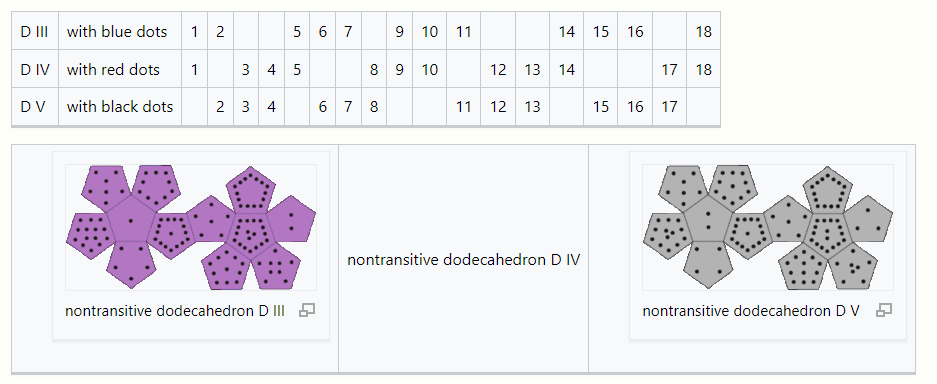Set 2: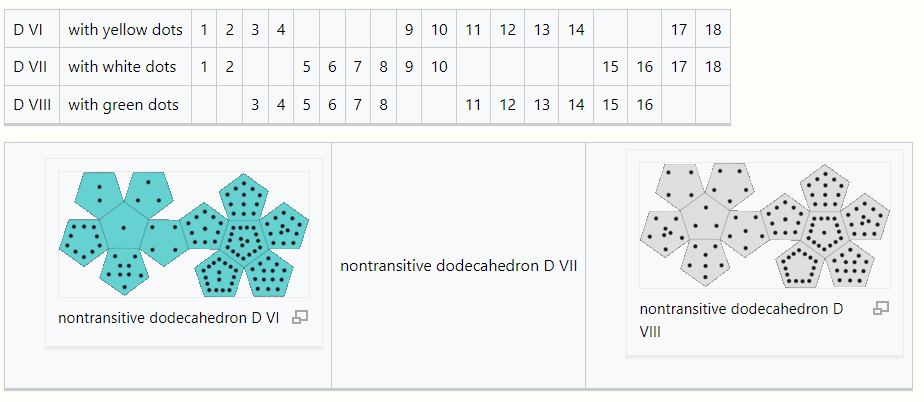### Nontransitive prime-numbered 12-sided dice

It is also possible to construct sets of nontransitive dodecahedra such that there are no repeated numbers and all numbers are primes. Miwin’s nontransitive prime-numbered dodecahedra win cyclically against each other in a ratio of 35:34.

Set 1: The numbers add up to 564.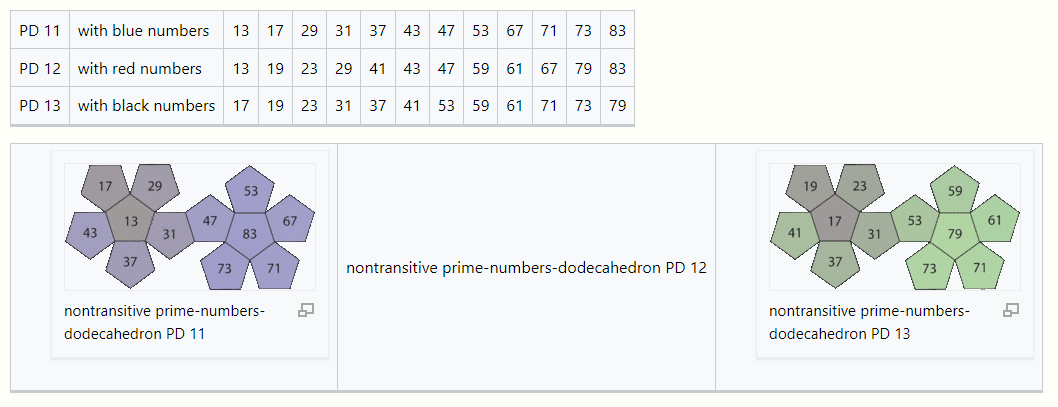Set 2: The numbers add up to 468.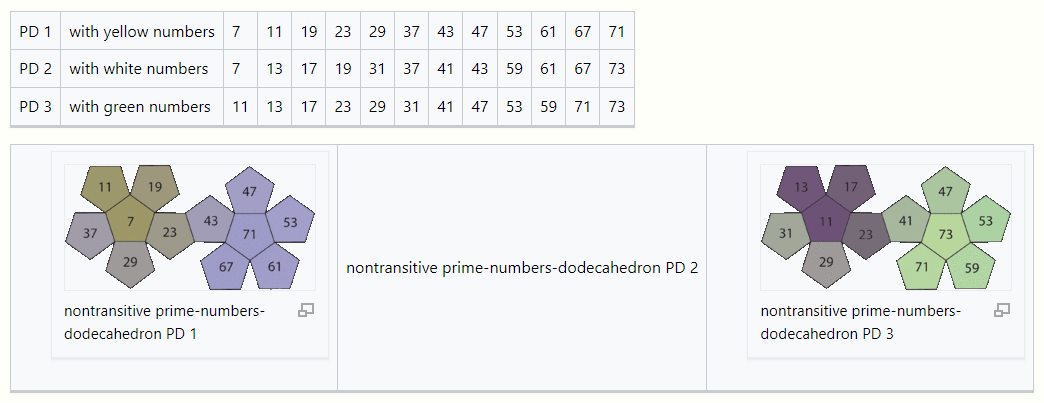### References

1. Bill Gates; Janet Lowe (1998-10-14). Bill Gates speaks: insight from the world's greatest entrepreneur. New York: Wiley. ISBN 9780471293538. https://books.google.com/books?id=Qoho6AIXTksC&pg=PA148&dq=Buffet+suggested+that+each+of+them+choose+one+of+the+dice,+then+discard+the+other+two.+They+would+bet+on+who+would+roll+the+highest+number+most+often.+Buffet+offered+to+let+Gates+pick+his+dice+first.+This+suggestion+instantly+aroused+Gates%27s+curiosity.+He+asked+to+examine+the+dice,+after+which+he+demanded+that+Buffet+choose+first. Retrieved 2011-11-29.
2. "like-a-marriage-only-more-enduring: Personal Finance News from Yahoo! Finance". Finance.yahoo.com. 2010-12-06. https://finance.yahoo.com/career-work/article/111491/like-a-marriage-only-more-enduring?mod=career-leadership. Retrieved 2011-11-29.
3. "Math Games - Tournament Dice by Ed Pegg Jr.". The Mathematical Association of America. 2005-07-11. http://www.mathpuzzle.com/MAA/39-Tournament%20Dice/mathgames_07_11_05.html. Retrieved 2012-07-06.
4. Nontransitive Dice ("Grime Dice") http://grime.s3-website-eu-west-1.amazonaws.com/
More
Information
Subjects:
Contributor MDPI registered users' name will be linked to their SciProfiles pages. To register with us, please refer to https://encyclopedia.pub/register :
View Times: 465
Entry Collection:
Revision: 1 time (View History)
Update Date: 28 Nov 2022
1000/1000